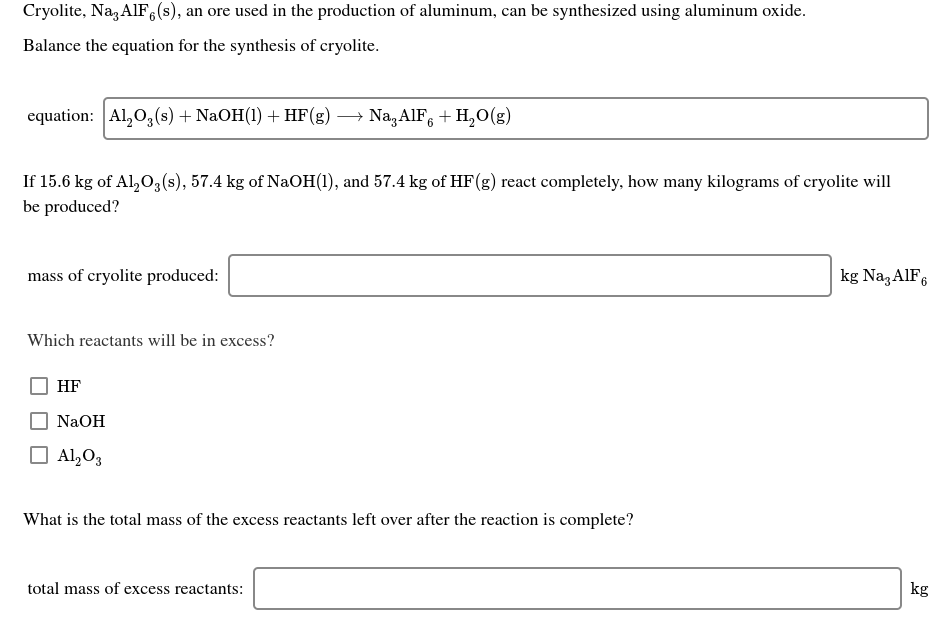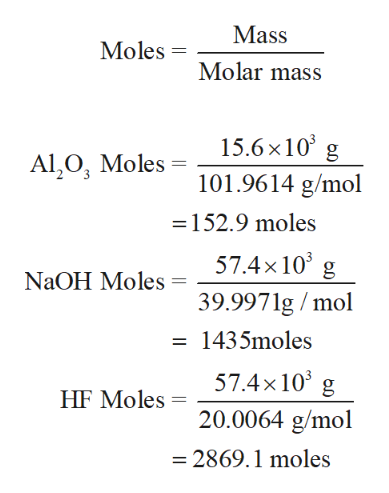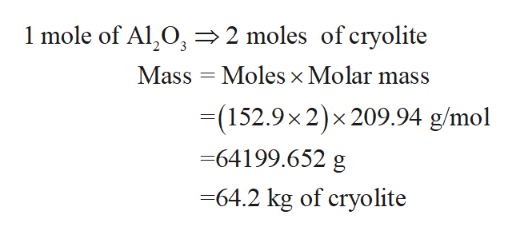# Cryolite, Na, AIF,(s), an ore used in the production of aluminum, can be synthesized using aluminum oxide.Balance the equation for the synthesis of cryolite.equation: Al,0,(s) + NaOH(1) + HF(g) → Na,AlF, + H,0(g)If 15.6 kg of Al,0,(s), 57.4 kg of NaOH(1), and 57.4 kg of HF(g) react completely, how many kilograms of cryolite willbe produced?kg Naz AIF6mass of cryolite produced:Which reactants will be in excess?HFNaOHO Al,O3What is the total mass of the excess reactants left over after the reaction is complete?total mass of excess reactants:kg

Question
8 viewshelp_outlineImage TranscriptioncloseCryolite, Na, AIF,(s), an ore used in the production of aluminum, can be synthesized using aluminum oxide. Balance the equation for the synthesis of cryolite. equation: Al,0,(s) + NaOH(1) + HF(g) → Na,AlF, + H,0(g) If 15.6 kg of Al,0,(s), 57.4 kg of NaOH(1), and 57.4 kg of HF(g) react completely, how many kilograms of cryolite will be produced? kg Naz AIF6 mass of cryolite produced: Which reactants will be in excess? HF NaOH O Al,O3 What is the total mass of the excess reactants left over after the reaction is complete? total mass of excess reactants: kg fullscreen
check_circle

Step 1

The balanced chemical reaction is as follows,

Step 2

Next, the given grams of reactant are converted into moles using mass and moles conversion.help_outlineImage TranscriptioncloseMass Moles Molar mass 15.6×10° g Al,O, Moles 101.9614 g/mol =152.9 moles 57.4x10° g NaOH Moles 39.9971g / mol = 1435moles 57.4×10° g HF Moles = 20.0064 g/mol = 2869.1 moles fullscreen
Step 3

Observing the balanced chemical equation clearly shows that 1 mole of Al2O3 requires 6 moles of NaOH and 12 moles of HF. Therefore, 152.9 moles of Al2O3 requires 917.4 NaOH and 1834.8 HF moles. Hence, the limtin...help_outlineImage Transcriptionclose1 mole of Al,0, =2 moles of cryolite Mass = Moles × Molar mass =(152.9x2)x 209.94 g/mol =64199.652 g =64.2 kg of cryolite fullscreen

### Want to see the full answer?

See Solution

#### Want to see this answer and more?

Solutions are written by subject experts who are available 24/7. Questions are typically answered within 1 hour.*

See Solution
*Response times may vary by subject and question.
Tagged in

### General Chemistry